BetMGM Championship Odds
+225
2.25 to 1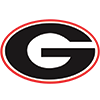Georgia
24.0% implied probability

+400
4 to 1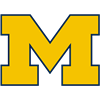Michigan
15.6% implied probability

+850
8.5 to 1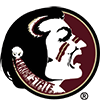Florida State
8.2% implied probability

+900
9 to 1Texas
7.8% implied probability

+900
9 to 1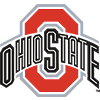Ohio State
7.8% implied probability

+1600
16 to 1USC
4.6% implied probability

+1600
16 to 1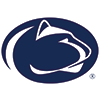Penn State
4.6% implied probability

+2000
20 to 1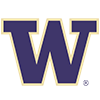Washington
3.7% implied probability

+2000
20 to 1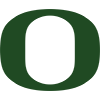Oregon
3.7% implied probability

+4000
40 to 1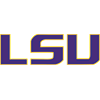LSU
1.9% implied probability

+4000
40 to 1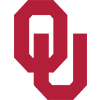Oklahoma
1.9% implied probability

+4000
40 to 1Notre Dame
1.9% implied probability

+5000
50 to 1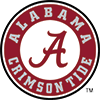Alabama
1.5% implied probability

+6000
60 to 1Utah
1.3% implied probability

+8000
80 to 1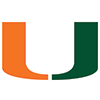Miami
1.0% implied probability

+12500
125 to 1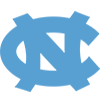North Carolina
0.6% implied probability

+12500
125 to 1Washington State
0.6% implied probability

+15000
150 to 1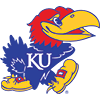Kansas
0.5% implied probability

+15000
150 to 1Duke
0.5% implied probability

+15000
150 to 1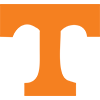Tennessee
0.5% implied probability

+15000
150 to 1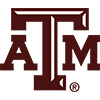Texas A&M
0.5% implied probability

+20000
200 to 1Clemson
0.4% implied probability

+20000
200 to 1Wisconsin
0.4% implied probability

+20000
200 to 1Missouri
0.4% implied probability

+25000
250 to 1Oregon State
0.3% implied probability

+25000
250 to 1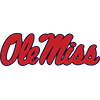Mississippi
0.3% implied probability

+25000
250 to 1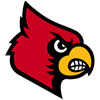Louisville
0.3% implied probability

+25000
250 to 1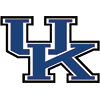Kentucky
0.3% implied probability

+25000
250 to 1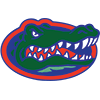Florida
0.3% implied probability

+30000
300 to 1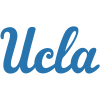UCLA
0.3% implied probability

+30000
300 to 1Kansas State
0.3% implied probability

+30000
300 to 1Iowa
0.3% implied probability

+35000
350 to 1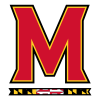Maryland
0.2% implied probability

+50000
500 to 1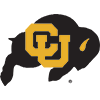Colorado
0.2% implied probability

+50000
500 to 1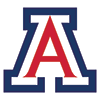Arizona
0.2% implied probability

+50000
500 to 1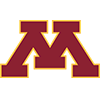Minnesota
0.2% implied probability

+50000
500 to 1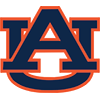Auburn
0.2% implied probability

+50000
500 to 1Wake Forest
0.2% implied probability

+50000
500 to 1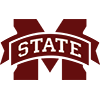Mississippi State
0.2% implied probability

+50000
500 to 1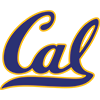California
0.2% implied probability

+50000
500 to 1Central Florida
0.2% implied probability

+50000
500 to 1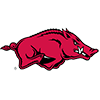Arkansas
0.2% implied probability

+50000
500 to 1North Carolina State
0.2% implied probability

+50000
500 to 1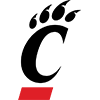Cincinnati
0.2% implied probability

+50000
500 to 1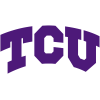TCU
0.2% implied probability

+50000
500 to 1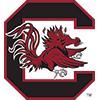South Carolina
0.2% implied probability

+50000
500 to 1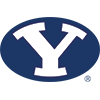BYU
0.2% implied probability

+100000
1000 to 1West Virginia
0.1% implied probability

+100000
1000 to 1Wyoming
0.1% implied probability

+100000
1000 to 1Vanderbilt
0.1% implied probability

+100000
1000 to 1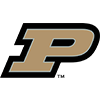Purdue
0.1% implied probability

+100000
1000 to 1Illinois
0.1% implied probability

+100000
1000 to 1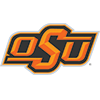Oklahoma State
0.1% implied probability

+100000
1000 to 1Arizona State
0.1% implied probability

+100000
1000 to 1Air Force
0.1% implied probability

+100000
1000 to 1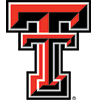Texas Tech
0.1% implied probability

+100000
1000 to 1Pittsburgh
0.1% implied probability

+100000
1000 to 1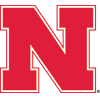Nebraska
0.1% implied probability

+100000
1000 to 1Michigan State
0.1% implied probability

+100000
1000 to 1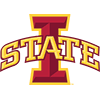Iowa State
0.1% implied probability

+100000
1000 to 1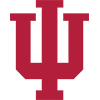Indiana
0.1% implied probability

+100000
1000 to 1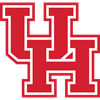Houston
0.1% implied probability

+100000
1000 to 1Coastal Carolina
0.1% implied probability

+100000
1000 to 1Baylor
0.1% implied probability

+100000
1000 to 1SMU
0.1% implied probability